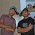INDEFINITE INTEGRATION NOTE BY PLANCESS

Indefinite integration, also known as antidifferentiation, is the reversing of the process of differentiation. Given a function f, one finds a function F such that F' = f.
Finding an antiderivative is an important process in calculus.

It is used as a method to obtain the area under a curve and to obtain many physical and electrical equations that scientists and engineers use everyday. For example, the equation for the current through a capacitor is , where I is current in Amperes, C is capacitance in Farads, V is voltage in Volts and t is time in seconds. To obtain an unknown (like V), one would have to use integration to obtain a voltage at a certain time interval.

While a true integral exists between a given boundary, taking the indefinite integral is simply reversing differentiation in much the same way division reverses multiplication. Instead of having a set of boundary values, one only finds an equation that would produce the integral due to differentiation without having to use the values to get a definite answer.

NOTE: jigssolanki.in does not own this book, neither created nor scanned. We just providing the link already available on internet. If any way it violates the law or has any issues then kindly mail us: jigssolanki1995@gmail.com or Contact Us for this(Link Removal).